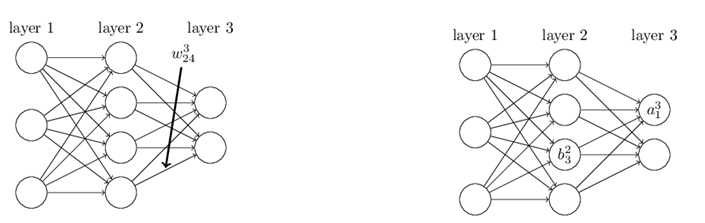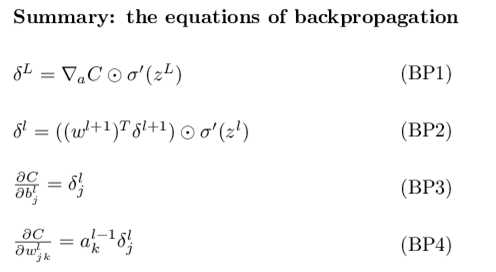# BP算法完整推导 2.0 (上)

### BP算法-变量声明

#### 回顾历史

BP 用来干嘛: 根据训练的误差, 来 动态 更新节点之间的 权值 .

BP算法相对于其他的神经网络学习算法会快很多, 这样就扩展了神经网络的解决问题的能力和领域. 直到目前, BP算法依然是深度学习的主要基石.

BP算法的核心是 求解误差函数 Loss 相对于权重 weights 和偏置 bias 的偏导, 来揭示 一旦改变 w, b 如何影响网络的整体行为.

#### 变量声明• $$w^l_{jk}$$ 第 l 层, 第 j 个节点, 到 第 $$(l-1)$$ 层的 第 k 个节点的 权值 (weight) ( 反向, 反向, 方向, 反向, 说了4遍)
• $$w^l$$ 第 l 层, 的 权值矩阵, 第 j 行, 第 k 列 为 $$w^l_{jk}$$
• $$b_j^l$$ 第 l 层, 第 j 个节点 的 bias (偏置)
• $$b^l$$ 第 l 层, 的 bias 向量
• $$a^l_j$$ 第 l 层, 第 j 个节点的 激励 (activation)
• $$a^l$$ 第 l 层, 的 激励 向量

$$z^l = w^l a^{l-1} + b^l$$

$$z^l$$ The weighted input to the neurons in layer l.

$$z^l_j =\sum \limits_k w_{jk} a_k ^{l-1} + b^l_j$$

$$a^l = \sigma(z^l)$$

• $$y = y(x)$$ 样本 x 的标签向量 (期望输出)

• $$a^L = a^L(x)$$ 样本 x 的网络输出激励向量
• n : 表示样本数量; L 表示网络层数

$$C = \frac {1}{2n} \sum \limits_x ||y(x) - a^L(x)||^2$$

#### 代价函数2大假设

$$C_x = \frac {1}{2} ||y - a^L||^2$$

$$C = \frac {1}{n} \sum\limits_x C_x$$

$$C = \frac{1}{2} ||y-a^L||^2 = \frac {1}{2} \sum\limits_j (y_j - a_j^L)^2$$

case:

$$(\alpha \odot \beta)_j = \alpha_j * \beta_j$$

$$[1, 2] \ \odot \ [3, 4] = [1*3, 2*4] = [3, 8]$$

### BP算法 - 四大公式

#### 理解 梯度的反方向

$$\sigma(z^l_j) \rightarrow \ \sigma(z^l_j + \Delta z^l_j)$$

• $$\frac {\partial C} {\partial z^l_j}$$ 非常大, 则表示 $$z^l_j$$ 对于整个网络的损失函数影响很大, 然后我们会选择与梯度符号相反的增量. (目的是让 "df" 接近 0, 接近函数 极值 的位置)
• $$\frac {\partial C} {\partial z^l_j}$$ 非常小, 则表示扰动的增量, 不论取何值, 对代价函数的影响很小, 则该神经元对于代价函数倾向于最优 , 也是是到达了函数的 局部极值点.

• 方向导数最大的方向, 为梯度方向, 其导数值就是梯度的模.
• 方向导数最小的放俩, 为梯度反方向, 其导数方向是梯度的摸

$$\delta ^l_j = \frac {\partial C}{\partial z^l_j}$$

tips: 算是第一遇见, 将导数值, 作为误差, 来训练, 这个脑洞, 真滴是可以的哦.

$$\frac {\partial C} {\partial w^l_{jk}}$$ , 以及 $$\frac {\partial C}{\partial b_j^l}$$BP算法完整推导 2.0 (上)

(0)
(0)